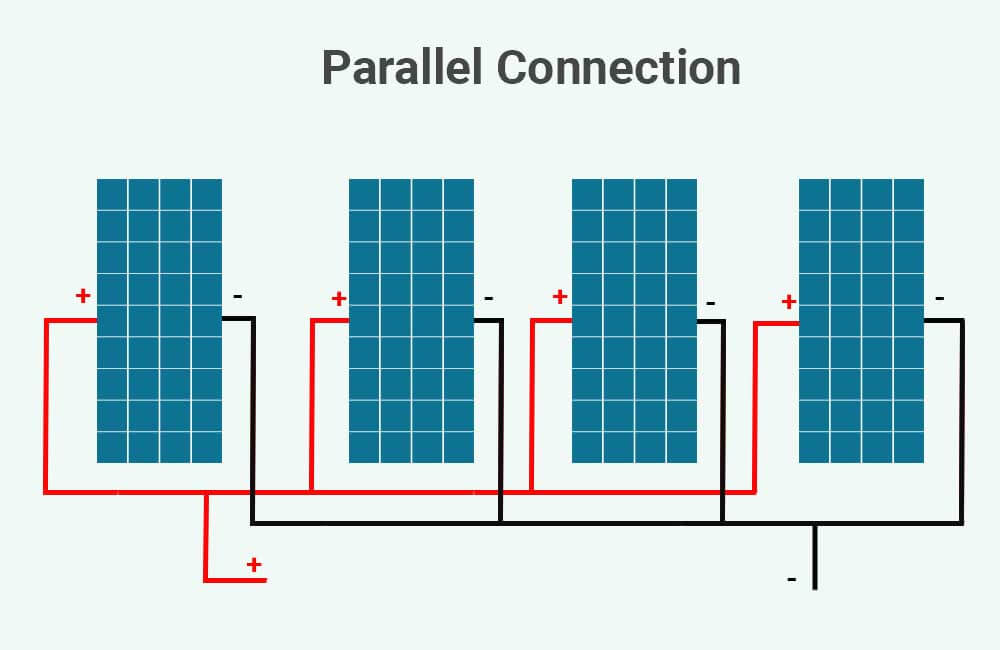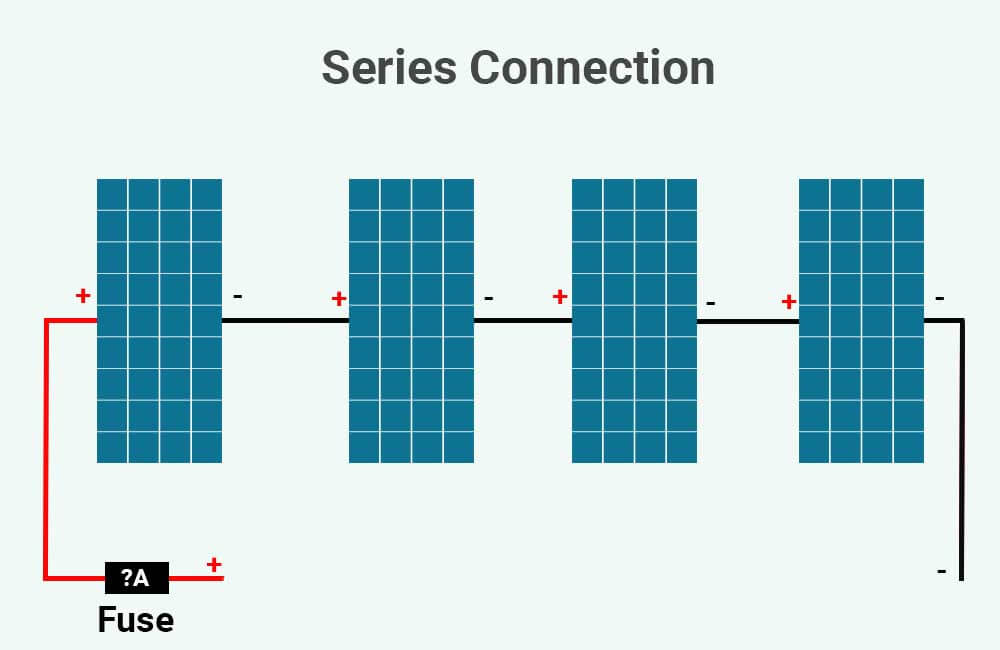# What size fuse between solar panel and charge controller?

Fuse and other overcurrent protection devices are an essential part of any electrical circuit.

In this article, I’ll explain why you need fuses between your solar panels and the solar charge controller, and I’ll show you how to correctly size these fuses.

## Do you need fuse between solar panel and charge controller?

The answer is yes, you do need a fuse between your solar panels and the charge controller. In fact, if there are parallel connections in your solar array, in addition to the fuse between your solar array and the charge controller, you’ll also need a fuse for each parallel string.

Should an overcurrent occur, a fuse (or fuses) between your panels and the charge controller would prevent your solar panels, charge controller, and wiring from overheating or even catching fire.

For example, let’s say we have 4 of these 200W solar panels from Renogy. And let’s also say that these solar panels are wired in parallel, as such:In their technical datasheet, Renogy specifies the following:

• Each of these solar panels has a short-circuit current of 9.66 Amps. Meaning that if a short circuit occurs, each of the solar panels would produce 9.66 Amps.
• The Maximum Series Fuse Rating for these panels is 15 Amps. Meaning that each of these solar panels should not operate at a current higher than 15 Amps.

Now, let’s imagine a scenario in which one of these panels shorts.

If a fault happens and one of the solar panels is short-circuited, its internal resistance will become negligible. Since electrical current flows in the least resistive available path, the current from all the solar panels will start flowing through the shorted solar panel.

So, the current flowing through the solar panel will be equal to the sum of the short-circuit currents from all 4 solar panels, which in this case is 38.64 Amps (4 x 9.66 Amps).

This amount of current exceeds the maximum 15 Amps specified by the manufacturer, which in turn would cause the solar panel to overheat and potentially sustain permanent damage or even catch fire.

If fuses (or overcurrent protection devices in general) were placed in each string, the shorted solar panel would be disconnected immediately.

In the next section, I provide more examples and explain how to size these fuses.

## What size fuse between solar panel and charge controller?

According to NEC’s Table 240.6 (A), the standard fuse sizes are: 10, 15, 20, 25, 30, 35, 40, 45, 50, 60, 70, 80, 90, 100, 110, 125, 150, 175, 200, 225, 250, 300, 350, 400, 450, 500, 600, 700, 800, 1000, 1200, 1600, 2000, 2500, 3000, 4000 5000, and 6000 Amps.

To determine the size of the fuse that you need between your solar array and the charge controller, multiply the total short-circuit current (in Amps) of your solar array by 1.56 and match that value to the equal, or next larger standard fuse amp rating.

As mentioned above, if you have parallel connections in your solar array, you’ll also need to fuse each string of solar panels. To determine the size of the fuse that you need for each string, multiply the short-circuit current of the string by 1.56 and match that value to the equal, or next larger standard fuse Amp rating.

In other words:

• If all of your solar panels are wired in series, you’ll only need 1 fuse, and you’ll only have to size 1 fuse.
• If you have parallel connections in your solar array, you’ll need 1 fuse between the solar array and the charge controller, and 1 fuse for each string. In this case, you’ll need to calculate 2 fuse sizes.

To understand this better, we’ll look at 3 different examples in which 4 solar panels are used, but in each example, the solar panels are wired in a different way.

For these examples, we’ll use the same 200W Renogy solar panels, which have a short-circuit current of 9.66 Amps.

### Example 1: Series connection

For this example, let’s say the solar panels are connected in series (positive to negative). Like this:When solar panels are connected in series, we call it a string. The total short-circuit current of the string will be equal to the short-circuit current of one solar panel, which in this case is 9.66 Amps.

Since we only have 1 string, we’ll only need 1 fuse.

Now, according to the NEC 690.9 (B), the fuse should be rated not less than 125% of the maximum current of the solar array.

But, what is the maximum current of the solar array?

According to the NEC 690.8 (A), the maximum current is the total short-circuit current of the solar array multiplied by 1.25. This is to account for cases where the amount of sunlight received by the solar panels exceeds the amount of sunlight the solar panels are rated for, which would mean a higher short-circuit current than the manufacturer specifies.

Since we have no parallel connections here, the total short-circuit current of the solar array is equal to the short-circuit current of one panel, which in this case is 9.66 Amps.

So, first, we’ll calculate the maximum current of the solar array:

Maximum Current (Amps) = Solar Array’s Short-circuit Current (Amps) x 1.25

Maximum Current (Amps) = 9.66 Amps x 1.25

Maximum Current (Amps) = 12.07 Amps

Now, we need to multiply this value by 1.25 (125%) to find the right size fuse:

Fuse size should be rated not less than = Maximum Current (Amps) x 1.25

Fuse size should be rated not less than = 12.07 Amps x 1.25

Fuse size should be rated not less than = 15 Amps

According to our calculations, we should use a 15A fuse in this case.

### Example 2: Parallel connection

For this example, let’s say the 4 solar panels are wired in parallel (positive to positive, negative to negative). Like this:Since we have 4 strings in parallel, we need 5 fuses in total. 1 fuse for each string (fuse 1, 2, 3, and 4), and 1 fuse for the solar array (fuse 5).

The 4 fuses in each string will have the same amp rating, which we can calculate using the same formulas:

Maximum Current (Amps) = Solar string’s Short-circuit Current (Amps) x 1.25

Maximum Current (Amps) = 9.66 Amps x 1.25

Maximum Current (Amps) = 12.07 Amps

We then multiply the max. current by 1.25 to find the fuse size:

Fuse size should be rated not less than = Maximum Current (Amps) x 1.25

Fuse size should be rated not less than = 12.07 Amps x 1.25

Fuse size should be rated not less than = 15 Amps

Again, the fuse size is 15 Amps. So we’ll need 4 15A fuses, one for each string.

Now, for the 5th fuse.

The total short-circuit current of the solar array is equal to the sum of the short-circuit currents of all the strings in parallel. Since each solar panel has a short-circuit current of 9.66 amps, the total short-circuit current of the array is 38.64 Amps (4 x 9.66 Amps).

Let’s multiply that by 1.25:

Maximum Current (Amps) = Solar Array’s Short-circuit Current (Amps) x 1.25

Maximum Current (Amps) = 38.64 Amps x 1.25

Maximum Current (Amps) = 48.3 Amps

And then multiply this value by 1.25:

Fuse size should be rated not less than = Maximum Current (Amps) x 1.25

Fuse size should be rated not less than = 48.3 Amps x 1.25

Fuse size should be rated not less than = 60 Amps

In this particular case, the fuse should be rated at 60 Amps.

### Example 3: Parallel/Series connection

For this example, we’ll assume the 4 solar panels are wired in a parallel/series configuration. Every 2 solar panels are wired in series, forming 2 strings, and then the 2 strings are wired in parallel.

Like this:Since we have 2 strings in parallel, we need 3 fuses in total. 1 fuse for each string (fuse 1 and 2), and 1 fuse for the solar array (fuse 3).

The 2 fuses in each string will have the same amp rating.

Let’s first calculate the maximum current:

Maximum Current (Amps) = Solar string’s Short-circuit Current (Amps) x 1.25

Maximum Current (Amps) = 9.66 Amps x 1.25

Maximum Current (Amps) = 12.07 Amps

And then multiply the max. current by 1.25:

Fuse size should be rated not less than = Maximum Current (Amps) x 1.25

Fuse size should be rated not less than = 12.07 Amps x 1.25

Fuse size should be rated not less than = 15 Amps

We’ll need 2 15A fuses, one for each string.

Now, for the 3rd fuse:

The total short-circuit current of the solar array is equal to the sum of the short-circuit currents of the 2 strings. Since each solar panel has a short-circuit current of 9.66 amps, the total short-circuit current of the array is 19.32 Amps (2 x 9.66 Amps).

Let’s multiply that by 1.25 to get the maximum current:

Maximum Current (Amps) = Solar Array’s Short-circuit Current (Amps) x 1.25

Maximum Current (Amps) = 19.32 Amps x 1.25

Maximum Current (Amps) = 24.15 Amps

And then multiply this value by 1.25:

Fuse size should be rated not less than = Maximum Current (Amps) x 1.25

Fuse size should be rated not less than = 24.15 Amps x 1.25

Fuse size should be rated not less than = 30 Amps

Fuse-3 should be rated at 30 Amps.

## Solar disconnect switch

According to the NEC 690.13, you’ll also be required to use a dual pole solar disconnect switch with ratings sufficient for the maximum current (total short-circuit current x 1.25) and the maximum voltage (total open-circuit voltage) from your solar array.

The switch should be placed before your solar charge controller and after your fuses and should be capable of disconnecting both the positive and the negative coming from your solar array.##### Younes

Hi! My name is Younes. I'm an electrical engineer and a renewable energy enthusiast. I created renewablewise.com with a mission of delivering digestible content and information to the people who seek it.

1. #### Val

Finally, the information I need given clearly and concisely. Thanks.

2. #### Lilly

Thank you so much for all the free information. I’ve used a bunch of your instructionals to calculate my needs in getting my solar system going. I’ve learned a lot from you and it’s very, very much appreciated.

3. #### JC S

I thought the sizing factor was 1.56. why are you only using 1.25 factor to size the fuse?

• #### Younes

Hello JC,
It’s 1.25 times the “Maximum Current”, which is equal to 1.25 time the Short-circuit Current (Isc). So the fuse size is actually 1.25 x 1.25 x Isc, which is 1.56 x Isc.
I hope this helps.